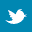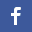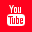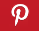Completely Solved C, C++ Programs Assignment.Quick Search Database Videos Tutorials Ebooks Forums FAQ Aboutus Household Industrial Manufacturing Service Shopping Transportation### C++ program to implement a Complex class that can store real part and imaginary part.

 Filed Under: C++ Assignments

Problem Statement :
Implement a COMPLEX class that can store real part and imaginary part with the following member functions / operators. One way to write a complex number is this: (3.0, 4.0). Here 3.0 is the real part and 4.0 is the imaginary part. Suppose a = (A,Bi) and c = (C,Di).Now do the following
(a) Constructor with default argument taken as real part = 0, imaginary part = 0
(b) Copy constructor
(c) Destructor
(d) Now overload the below operators
i) = i.e. a = c
ii) + i.e. a + c = (A + C, (B + D)i)
iii) - i.e. a – c = (A – C, (B – D)i)
iv) * i.e. a &#61655;&#61472;c = (A &#61655;&#61472;C – B &#61655;&#61472;D, (A &#61655;&#61472;D + B &#61655;&#61472;C)i)
v) * i.e. (x a real number): x &#61655;&#61472;c = (x &#61655;&#61472;C, x &#61655;&#61472;Di)
vi) ~ (Conjugation) i.e. ~a = (A, – Bi)
vii ) >> and << to take input and output complex numbers
(e) Also overload these operators +=, = =, >, ++(pre), ++(post)
(f) cast operator to int (i.e. get the real part exported)

Logical Approach :
The above program is done using an Abstract Data Type (ADT) called class. A class is a mechanism to create objects. In this program a class named complex is created.
It contains the following data members :
• real(denoting real part of a complex number)
• imaginary (denoting imaginary part of a complex number )
It contains the following member functions :
• complex() -Default constructor.
• complex(double,double) -Parameterized constructor, used to initialize a complex number.
• complex(const complex&) -Copy constructor, used to make a new copy of a complex number.
• operator double()-Used to cast operator to int convertion(real part of a complex number is extracted).
• operator+(complex)-Used to add two complex numbers.
• operator-(complex)-Used to subtract two complex numbers.
• operator*(complex)-Used to multiply two complex numbers.
• operator/(complex)-Used to divide two complex numbers.
• operator*(double)-Used to multiply a complex number with a scalar value.
• operator++()-Used to increment(pre) a complex number by 1.
• operator++(int)-Used to increment(post) a complex number by 1.
• operator+=(complex)-Used to add a complex number passed as parameter to the original complex number,original complex number will be modified.
• operator==(complex)-Used to check equality of two complex numbers.
• operator>(complex)- Used to check whether a complex number is greater than another.
• operator<<(ostream&,complex&)-Overload <<(cout) operator which displays a complex number.
• operator>>(istream&,complex&)- Overload >>(cin) operator which takes a complex number as input.
• operator=(complex)-Used to assign a complex number to another.
• operator~()-Used to find conjugate of a complex number.

In this program we have used operator overloading feature. Operator overloading (less commonly known as operator ad-hoc polymorphism) is a specific case of polymorphism in which some or all of operators like +, =, or == have different implementations depending on the types of their arguments. Sometimes the overloadings are defined by the language; sometimes the programmer can implement support for new types.
To define an additional task to an operator,we must specify what it means in relation to the class to which the operator is applied. This is done with help of an operator function,which describes the task. Operator functions must be either member functions(non-static) or friend functions. In this program,we have done all the operations by using member functions except overloading of << and >> operators. These two operators are overloaded using friend functions.
First user should enter two complex numbers on which he or she wants to perform some arithmetic operations and visualize the result on the screen. After performing several operations mentioned above user can exit from the program, can continue operation with previous two fractions or can continue operation after taking another two complex numbers as input; choice is totally up to user.

Algorithm :
Creating an ADT with name complex

Step 2:Declaring private data members
int real//Declaring an integer
int imaginary//Declaring an integer

Step 3:Declaring public member functions
complex();
complex(double,double);
complex(const complex&);
operator double();
operator+(complex);
operator-(complex);
operator*(complex);
operator*(double);
operator/(complex);
operator=(complex);
operator~();
operator++();
operator++(int);
operator+=(complex);
operator==(complex);
operator>(complex);
operator<<(ostream&,complex&);
operator>>(istream&,complex&);
};

Default constructor

complex()
{
Initializing real and imaginary as 0;
}

Parameterized constructor

complex(real, imaginary)
{
Initializing real and imaginary with the values passed as parameters;
}
Copy constructor

complex(const complex &cmp)
{
real=cmp.real;
imaginary=cmp.imaginary;
}

This function is used to add two complex numbers

Procedure operator+(complex num1)
{

real=num1.real+real;
imaginary=num1.imaginary+imaginary;
complex num3(real,imaginary);
}

This function is used to subtract two complex numbers

Procedure operator-(complex num1)
{

real=num1.real-real;
imaginary=num1.imaginary-imaginary;
complex num3(real,imaginary);
}

This function is used to multiply two complex numbers

Procedure operator*(complex num1)
{

real=num1.real*real-num1.imaginary*imaginary;
imaginary=num1.real*imaginary+num1.imaginary*real;
complex num3(real,imaginary);
}

This function is used to divide two complex numbers

Procedure operator/(complex num1)
{
tmp=num1.real*num1.real+num1.imaginary*num1.imaginary;
real=(num1.real*real+num1.imaginary*imaginary)/tmp;
imaginary=(num1.real*imaginary-num1.imaginary*real)/tmp;
complex num3(real,imaginary);
return num3;
}

This function is used to multiply a complex number with a scalar value

Procedure operator*(num1)
{
real=num1*real;
imaginary=num1*imaginary;
complex num3(real,imaginary);
}

This function is used to cast operator to int convertion(real part of a complex number is extracted)

Procedure operator double()
{
return real;
}

This function is used to assign a complex number to another

Procedure operator=(complex cmp)
{
real=cmp.real;
imaginary=cmp.imaginary;
}

This function is used to find conjugate of a complex number

Procedure operator~()
{
real=complex::real;
imaginary=-complex::imaginary;
complex num3(real,imaginary);
}

This function is used to increment(pre) a complex number by 1

Procedure operator++()
{
++real;

}

This function is used to increment(post) a complex number by 1

Procedure operator++(x)
{
tmp=real;
real++;
return tmp;
}

This function is used to add a complex number passed as parameter to the original
complex number,original complex number will be modified

Procedure operator+=(complex cmp)
{
real+=cmp.real;
imaginary+=cmp.imaginary;
}

This function is used to check equality of two complex numbers

Procedure operator==(complex c)
{
if(real==c.real)
{
if(imaginary==c.imaginary)
return true;
}
return false;

}

This function is used to check whether a complex number is greater than another

Procedure operator>(complex c)
{
c1= real*real+imaginary*imaginary;
c2=c.real*c.real+c.imaginary*c.imaginary;
if(c1>c2)
return true;
return false;
}

Overload <<(cout) operator which displays a complex number

Procedure operator<<(ostream &dout,complex &c)
{
if(c.imaginary>=0)
dout<<c.real<<"+"<<c.imaginary<<"i\n";
else
dout<<c.real<<c.imaginary<<"i\n";
return (dout);
}

Overload >>(cin) operator which takes a complex number as input

Procedure operator>>(istream &din,complex &c)
{
din>>c.real;
din>>c.imaginary;
return (din);
}

Main procedure

do
{
complex num1,num2;
read 1st and 2nd complex number in num1 and num2;
complex num3,num4;
num3=num1+num2;
if(num1==num2)
Print “Two complex numbers are equal.";
if(num1>num2)
Print "1st complex number is greater than 2nd complex number.";
if(num2>num1)
Print “2nd complex number is greater than 1st complex number.";
Print "The result of addition is: ";
Print num3;
num3=num1-num2;
Print “The result of subtraction is: ";
Print num3;
num3=num1*num2;
Print “result of multiplication is: ";
Print num3;
num3=num1/num2;
Print "The result of division is: ";
Print num3;
Read a scaler value in sc";
Print "The result of scaler multiplication";
Print "For 1st complex number:";
num3=num1*sc;
Print num3;
Print "For 2nd complex number:";
num3=num2*sc;
Print num3;
Print "Conjugate of 1st complex number:";
num3=~num1;
Print num3;
Print "Conjugate of 2nd complex number:";
num3=~num2;
Print num3;
Print "Complex Number assignment";
Print "For 1st complex number:";
num4=num3=num1;
Print num3;
Print num4;
Print "For 2nd complex number:";
num4=num3=num2;
Print num3;
Print num4;
Print "Casting 1st complex number:";
cast=num1;
Print cast;
Print "Casting 2nd complex number:";
cast=num2;
Print cast;
Print "Copy using copy constructor\n";
Print "Copy of 1st complex number:";
complex num5=num1;
Print num5;
Print "Copy of 2nd complex number:";
complex num6=num2;
Print num6;
Print "After Increment(++) operation(pre) on 1st complex number we get:";
++num1;
Print num1;
Print "After Increment(++) operation(post) on 2nd complex number we get:";
num2++;
Print num2;
Read another complex number in num3;
num1+=num3;
Print num1;
num2+=num3;
Print num2;
Print "Do you want to check for another two complex numbers(Y/N):";
}while(ch=='Y'||ch=='y');

Discussions :
&#61548; Namespaces allow to group entities like classes, objects and functions under a name. This way the global scope can be divided in "sub-scopes", each one with its own name.

&#61548; All the files in the C++ standard library declare all of its entities within the std namespace. That is why we have generally included the using namespace std; statement in all programs that used any entity defined in iostream.

&#61548; We have used new for dynamically instanciate num and deno.

&#61548; A copy constructor is a special constructor in the C++ programming language used to create a new object as a copy of an existing object. The first argument of such a constructor is a reference to an object of the same type as is being constructed (const or not), which might be followed by parameters of any type (all having default values).

&#61548; A shallow copy of an object copies all of the member field values. This works well if the fields are values, but may not be what you want for fields that point to dynamically allocated memory. The pointer will be copied. but the memory it points to will not be copied -- the field in both the original object and the copy will then point to the same dynamically allocated memory, which is not usually what you want. The default copy constructor and assignment operator make shallow copies.
A deep copy copies all fields, and makes copies of dynamically allocated memory pointed to by the fields. To make a deep copy, you must write a copy constructor and overload the assignment operator, otherwise the copy will point to the original, with disasterous consequences. Here we have used shallow copy method in this program.

&#61548; The general form of an operator function is,
&#61548; return type classname :: operator op(arglist)
{Back to main directory:Software Practical